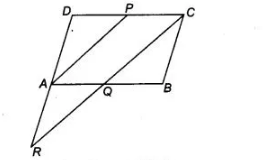# P is the mid-point of the side CD of a parallelogram ABCD.`
Question:

P is the mid-point of the side CD of a parallelogram ABCD. A line through C parallel to PA intersects AB at Q and DA produced at R. Prove that DA = AR and CQ = QR.

Solution:

Given in a parallelogram $A B C D, P$ is the mid-point of $D C$.

To prove $D A=A R$ and $C Q=Q R$

Proof $A B C D$ is a parallelogram.$\therefore$ $B C=A D$ and $B C \| A D$

Also, $D C=A B$ and $D C \| A B$

Since, $P$ is the mid-point of $D C$.

$\therefore$ $D P=P C=\frac{1}{2} D C$

Now, $Q C \| A P$ and $P C \| A Q$

So, $A P C Q$ is a parallelogram.

$\therefore$ $A Q=P C=\frac{1}{2} D C$

Now, $Q C \| A P$ and $P C \| A Q$

So, $A P C Q$ is a parallelogram.

$\therefore \quad A Q=P C=\frac{1}{2} D C$

$=\frac{1}{2} A B=B Q$ $[\because D C=A B] \ldots(i)$

Now, in $\triangle A Q R$ and $\triangle B Q C$. $A Q=B Q$ [from Eq. (i)]

$\angle A Q R=\angle B Q C$ [vertically opposite angles]

and $\quad \angle A R Q=\angle B C Q \quad$ [Alternate interior angles]

$\therefore$ $\triangle A Q R \equiv \triangle B Q C$ [by AAS congruence rule]

$\therefore$ $A R=B C$ [by CPCT rule]

But $B C=D A$

$\therefore \quad A R=D A$

Also, $C Q=Q R$ [by CPCT rule]

Hence proved.